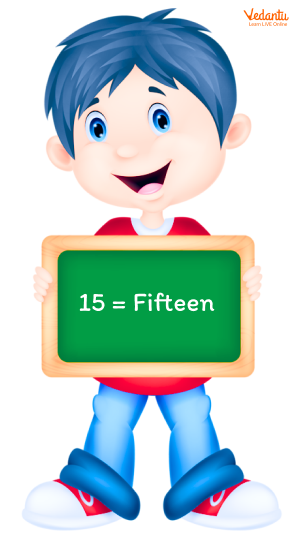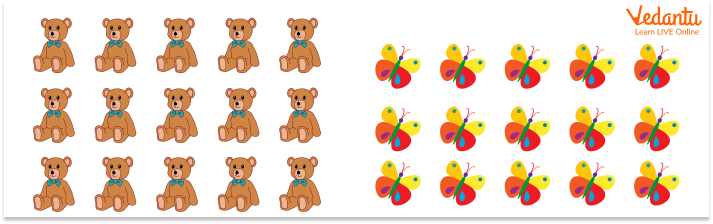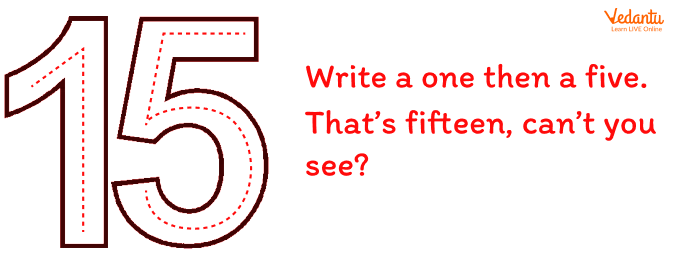Courses
Courses for Kids
Free study material
Free LIVE classes
More

# Introduction to the Number 15 to Kids## What is a Number?

Before discussing the number fifteen and its properties, let us understand what a number is. A number is defined as the arithmetic value of a quantity. Numbers are used in our everyday lives. They are often called numerals. The world as we know it today would not have progressed if we lacked the understanding of numbers. Without numbers, we could not count things, dates, time, money, etc. The use of numbers is not limited to this, they are used to carry out complex arithmetic and mathematical operations. The article introduces kids to the number 15. It also provides some fun ways which can help kids memorise numbers.

## What is the Number 15?

The number 15 is defined as the Mathematical representation of the value or quantity of the fifteen number. 15 is preceded by the number 14 and is succeeded by the number 16. 15 is a whole number.

## How to Write 15 in Words?

Let us now look at how to write 15 in words.

• 15 can be written as fifteen.

• Fifteen number is mathematically represented as 15.Number 15 Written in Words

## How to Count the Fifteen Number?

The counting of numbers starts from 1. One is the first whole number. 1 is followed by 2, 3, 4, 5, 6, 7, 8, 9, 10, 11, 12, 13, 14, and then 15. The counting till the fifteen number can be better understood by the example shown below.15 Toys and 15 Butterflies

The image illustrated shows that there are 15 teddy bears and there are 15 butterflies. There are various other examples like:

• There are 15 monkeys in a zoo.

• There are 15 apples in a basket.

• There are fifteen candies in a packet.

## Fun Way to Learn the Number Fifteen

Parents can teach kids about the number 15 by incorporating it in some songs and rhymes. Another fun way of learning what 15 is to engage kids by counting till 15, one can ask them how many candies are in a packet. Mentioned below is a small poem that can help kids to learn how to write the number 15.How to Write the Number Fifteen?

Mentioned below is a small rhyme that can help teachers and parents to familiarise kids with the number fifteen.

Fifteen little girls,

Dancing on their toes!

Stood on fifteen chairs,

In a ballerina pose!

## Conclusion

We have learnt about 15, the number. We have also learnt how to count to 15 and write 15 in English. As children progress to senior grades, they are exposed to a variety of concepts. Teaching children using graphics and entertaining activities, as described in the article, can help them grasp more. We hope that the article stimulates children's interest in numbers and Mathematics.

Last updated date: 24th Sep 2023
Total views: 127.2k
Views today: 2.27k

## FAQs on Introduction to the Number 15 to Kids

1. How to teach kids to learn the number 15?

Teaching kids how to write a number can be a fun experience for both parents and kids. Kids frequently love vivid sheets and graphics. Printing colourful papers with dots that approximate the number is an option for parents. Teach children to connect the dots so that they can become familiar with the number's structure. Children can be asked to count or observe objects in their environment that are fifteen in number. Instead of giving your child number worksheets, you might talk about other facts about the number fifteen, such as the number of minutes in a quarter-hour.

2. Is 15 a cardinal number?

Yes, 15 is a cardinal number. Cardinal numbers are defined as numbers that are used to count things. Cardinals are also referred to as natural numbers. The cardinal numbers are the counting numbers that start from 1 and go on till infinity. Examples of cardinal numbers include one, two, three, four, and so on. An important point to note is that cardinal numbers do not include fractions or decimals.